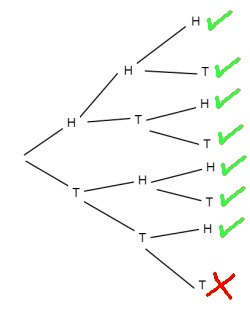If Busi has 3 children what is the probability that at least one will be a girl?

Imagine tossing a coin three times.
What’s the probability you will get a head on at least one of the tosses?

Suppose you drew this tree diagram. How would it help you to find the answers to these questions?What is the connection between the questions?

How could you work out the probability of Busi having 3 boys?

What do you notice about the probabilities of having at least one girl and having 3 boys?

Thank you to NRICH for the idea for this problem. You will find similar problems on the NRICH website.